Publication date: 11/29/2021

## Power for Two Independent Sample Variances

Use the Power Explorer for Two Independent Sample Variances to determine a sample size for a hypothesis test for variances from two groups. Select DOE > Sample Size Explorers > Power > Power for Two Independent Sample Variances. Explore the trade offs between sample size, power, significance, and the hypothesized difference to detect. Sample size and power are associated with the following hypothesis test: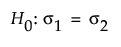versus the two-sided alternative: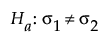or versus a one-sided alternative: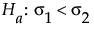or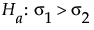where σ1 is the variance for Group 1 and σ2 is the variance for Group 2. The difference to detect is an amount away from σ2 that one considers as important to detect based on a set of samples. This difference is expressed as the ratio of σ2/σ1, or the ratio of your variances. For the same significance level and power, a larger sample size is needed to detect a small difference in variances than to detect a large difference. It is assumed that the populations of interest are normally distributed.

### Power Explorer for Two Independent Sample Variances Options

Set study assumptions and explore sample sizes using the radio buttons, text boxes, and sliders. The curve updates as you make changes to the settings. Alternatively, change settings by dragging the cross hairs on the curve or adjusting the values in the axis text boxes.

Test Type

Specifies a one or two-sided hypothesis test.

Fixed Parameters

Alpha

Specifies the probability of a type I error, which is the probability of rejecting the null hypothesis when it is true. It is commonly referred to as the significance level of the test. The default alpha level is 0.05.

Test Parameters

Parameters that are inter-related and update as you make changes.

Ratio of variances (Group 2/Group 1)

Specifies the ratio of the variances.

Group 1 Sample Size

Specifies the number of observations (runs, experimental units, or samples) needed for Group 1 in your experiment. Select Lock to lock the Group 1 sample size.

Group 2 Sample Size

Specifies the number of observations (runs, experimental units, or samples) needed for Group 2 in your experiment. Select Lock to lock the Group 2 sample size.

Total Sample Size

Specifies the total number of observations (runs, experimental units, or samples) needed for your experiment. The power curve is based on total sample size. Select Lock to lock the total sample size.

Note: Adjusting the sample size for one group adjusts the total sample size unless the total sample size is locked. In that case, adjusting the sample size for one group adjust the sample size for the second group. Adjusting the total sample size adjusts the two groups proportionally, unless one group is locked. Use only one lock at a time.

Power

Specifies the probability of rejecting the null hypothesis when it is false. With all other parameters fixed, power increases as sample size increases.

### Statistical Details for the Two Independent Sample Variances Explorer

The power calculations for testing the ratio of variances from two sample groups is based on the standard F test. The calculations depend on the form of the alternative hypothesis. For a one-sided, higher alternative: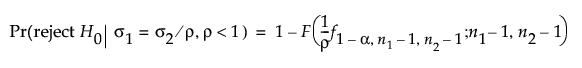For a one-sided, lower alternative: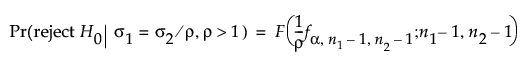For a two-sided alternative: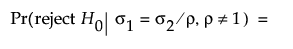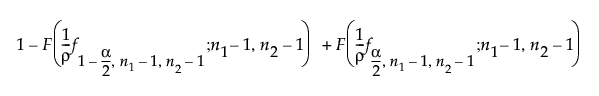where:

α is the significance level

n1 and n2 are the group sample sizes

ρ = σ2/σ1

f1-α,ν1,ν2 is the (1 - α)th quantile of an F distribution with ν1 and ν2 degrees of freedom

F(x, ν) is the cumulative distribution function of an F distribution with ν degrees of freedom.